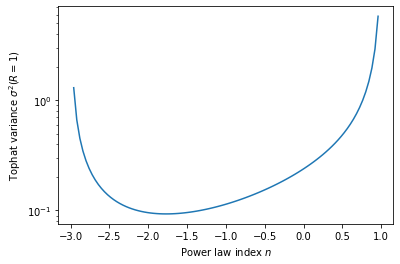# $\sigma_8$ in power-law cosmologies¶

Author: Lehman Garrison (https://lgarrison.github.io)

#### Update 10/25/2019:¶

Thanks to Daniel Eisenstein for deriving an even simpler expression! The notebook has been updated with this version.

Power-law cosmologies ($P(k) = A k^n$) have an analytic form for $\sigma^2(R)$ with a spherical tophat window $W_T$:

\begin{align} \sigma^2(R) &= \int \frac{d^3k}{(2\pi)^3}\tilde W_T^2(kR)P(k) \\ &= A \int_0^\infty \frac{k^2dk}{2\pi^2} \left[\frac{3(\sin(kR) - kR\cos(kR))}{(kR)^3}\right]^2 k^n\\ &= \frac{9 A (n+1) 2^{-n-1} R^{-n-3} \sin \left(\frac{\pi n}{2}\right) \Gamma (n-1)}{\pi^2 (n-3)} \end{align}

where we used the Fourier transform $\tilde W_T(kR)$ of the spherical tophat window. The result in the last line (obtained via Mathematica) is only valid for $-3 < n < 1$; otherwise, the variance is undefined.

However, the result is numerically unstable if $n$ is an integer in this range, since the function $\Gamma(n-1)$ in the numerator goes to infinity for 0 and the negative integers.

The $\sigma^2(R)$ function itself is perfectly smooth and finite in this range, as seen below:

In :
import numpy as np
from scipy.special import gamma
def sigma2_mathematica(n,R):
return 9*(n + 1)*2**(-n - 1)*R**(-n - 3)*np.sin(np.pi*n/2)*gamma(n - 1)/(np.pi**2*(n-3))

%matplotlib inline
import matplotlib.pyplot as plt
nrange = np.linspace(-3,1,101, endpoint=False)
plt.plot(nrange, sigma2_mathematica(nrange,1))
plt.xlabel('Power law index $n$')
plt.ylabel('Tophat variance $\sigma^2(R=1)$');
plt.yscale('log')

plt.savefig('scale_free_sigma8.pdf')For plotting purposes, we avoided exact integers in the $n$ range and used $A=1$.

So, to find a numerically stable variant of the above expression, we need to re-write it to avoid evaluating $\Gamma(n-1)$ at negative integers.

Using some properties of the $\Gamma$ function (https://dlmf.nist.gov/5.5), we can arrive at the following expression:

$$\sigma^2(R) = \frac{9 A R^{-(3+n)}}{2 \pi^{3/2}} \frac{\Gamma[(3+n)/2]}{\Gamma[(2-n)/2] (n-3)(n-1)}$$

It's worth noting that this expression is exact; we have not used any approximations.

Note: a previous version of this notebook had two expressions, one for even poles and one for odd, which are now combined into one expression.

In :
def sigma2_robust(n,R):
return 9*R**-(3 + n)/(2*np.pi**(3/2)) * gamma((3 + n)/2)/(gamma((2 - n)/2)*(n - 3)*(n - 1))

In :
import pandas as pd

def test_all():
n = np.array([-2.9, -2.5, -2, -1.3, -1, -0.01, 0, 0.001, 0.75])
R = 8.

res = pd.DataFrame(index=n)
res.index.name = 'n'
res['Naive'] = sigma2_mathematica(n, R)
res['Stable'] = sigma2_robust(n, R)

return res

In :
res = test_all()
res

/home/lgarrison/anaconda3/lib/python3.7/site-packages/ipykernel_launcher.py:4: RuntimeWarning: invalid value encountered in multiply
after removing the cwd from sys.path.

Out:
Naive Stable
n
-2.900 0.432508 0.432508
-2.500 0.047497 0.047497
-2.000 -inf 0.011937
-1.300 0.002945 0.002945
-1.000 NaN 0.001781
-0.010 0.000471 0.000471
0.000 NaN 0.000466
0.001 0.000466 0.000466
0.750 0.000392 0.000392
In :
print(res.to_latex(float_format=lambda s:'{:.6g}'.format(s)))

\begin{tabular}{lrr}
\toprule
{} &       Naive &      Stable \\
n      &             &             \\
\midrule
-2.900 &    0.432508 &    0.432508 \\
-2.500 &   0.0474965 &   0.0474965 \\
-2.000 &        -inf &   0.0119366 \\
-1.300 &  0.00294465 &  0.00294465 \\
-1.000 &         nan &  0.00178104 \\
-0.010 &  0.00047106 &  0.00047106 \\
0.000 &         nan & 0.000466274 \\
0.001 & 0.000465801 & 0.000465801 \\
0.750 & 0.000392073 & 0.000392073 \\
\bottomrule
\end{tabular}


In [ ]: